# Python Operators — Certainly Not Very Interesting, But Very Important

## They may seem trivial at first glance, but believe me, they really fast get crucial

In my last article, we covered the basics, how to start coding with Python. We learned how to set up a programming environment. Also, we encountered data types, variables, and data structures. With this article we will continue our journey, today we will learn what useful thing we can do with variables or data structures.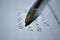Photo by Antoine Dautry on Unsplash

The article is structured as follows:

Enjoy the reading!

# Introduction

Just like when we first started with math, we learned about numbers, and then, we started to do something with those numbers, so-called operations, like addition, multiplication, or comparison. Similarly, to make some use of Python overall and the knowledge from the previous article, we will today introduce operators.

In Python, operators are special characters, which will seem very familiar. Also, it’s important to define operands, the numbers or variables on which the operations are conducted.

Basically, we have two operands, the variables, numbers, string on the left and right, and the operator in the middle of them.

Before we start, let’s open a Jupyter Notebook. If you forgot how, check the last article. 🙂

# Arithmetic operators

Arithmetic operators are used for basic mathematical operations, i.e. addition, subtraction, multiplication, division, modulo operation (modulus), exponentiation, and floor division.

Let’s start with addition. Its operator is the plus sign +. It adds the numbers on either side of the sign.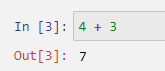by author

Subtraction is done using the minus sign -. It subtracts the right-hand operand from the left-hand operand.by author

Multiplication is done using the asterisk sign *. It multiplies values on either side of the operator.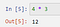by author

Division is done using the forward-slash /. It divides the left-hand operand by right-hand operand. It’s important to note that even when two integers are divided, the operation returns a float.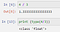by author

The modulo operation or modulus divides the left-hand operand by the right-hand operand and returns the remainder. It’s done using the percent sign %.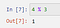by author

Exponentiation or power operation performs exponential (power) calculation on operators. It can be thought of as repeated multiplication. It’s done using two asterisk signs **.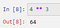by author

Last but not least, we have floor division. It’s a division of operands where the result is the quotient in which the digits after the decimal point are removed.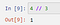by author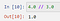by author

If we input two integers, the output is also an integer, while using floats returns a float.

All these examples can also be done using variables. Here’s an example of multiplication.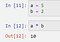by author

Now we covered the basics of arithmetic operators, we will meet them later, in more complex operations. But let’s now see another important group of operators.

# Comparison Operators

As the name suggests, they compare the values on either side of them and decide the relation among them. They are also called Relational operators. They return a Boolean value, or in other words, True or False. Let’s jump into some examples. We will use variables now, a will hold 14 and b will hold 23.

The equal operator checks if the two variables (or numbers) are equal. It checked by using two equal signs ==.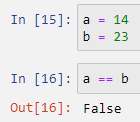by author

If the values of two operands are equal, then the condition becomes true. Since a and b are not equal it returns the Boolean value False. This means that the not equal operator should return True. It’s checked by using an exclamation mark and equal sign !=.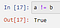by author

To check if a is greater than b we use the greater than operator >. It checks if the value of the left operand is greater than the value of the right operand, then the condition becomes True.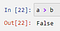by author

Since a is less than b, it returns False.

To prove that a is smaller than b, let’s use the less-than operator <. If the value of the left operand is less than the value of the right operand, then condition becomes True.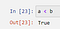by author

Since 14 is lower than 23, it returns True.

In addition to greater than and less than, there are greater than or equal to and less than or equal to operators, checked with >= or <= signs, respectively. Greater than or equal to returns True if the value of the left operand is greater than or equal to the value of the right operand.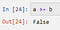by author

If the left-hand operand is smaller than the right-hand operand the less than or equal to, returns true.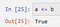by author

Comparison operators will often be used to check if certain conditions are met, for example in loops which will be covered later.

# Assignment Operators

Assignment operators are used in cases where we want to assign a value to a variable. We have already encountered the first assignment operator, the equal sign =. It is used when a constant (value) is assigned to a variable.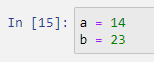by author

Or we want to assign the sum of two variables, a and b, to a new variable, c.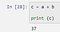by author

Or, shorter written.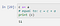by author

Similarly, we can do any other arithmetic operation mentioned above, combined with an assignment, subtraction, multiplication, division, modulo, exponentiation, and/or floor division. Only multiplication is presented, but any other can be done following the example.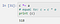by author

First, c is multiplied by a, and then the new value, 518, is assigned to c.

For all the mentioned operation, take the left operand, add, subtract, multiply… it with the right operand, and assign the result to the left operand.

# Logical Operators

Logical operators are used in cases where we want to combine conditional statements. I.e., we want to check if two or more conditions are met. There are three logical operators, and, or and not.

The and-operator returns True if all checked conditions are True. If just one condition is not met, it will return False.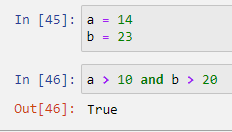by author

The or operator will return True if at least one checked condition is True. It will only return False when none of the conditions is met.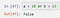by author

Here, both conditions are False, hence the or operator returned False.

The not operator returns True if all conditions are False, or it returns False, if all conditions are True. Or easily, we can think of it, if we wanted the opposite of the and operator.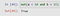by author

There are a lot more combinations possible, and I highly encourage you to try them by yourself, since it sometimes can get confusing, and the best way is to try it out.

# Bitwise Operators

Bitwise operators are used when we want to compare numbers on the binary level. The integers are first converted to binary format and then the selected operation is performed bit by bit. The result is returned in decimal format. The bitwise operators are & (and), | (or), ^ (xor), ~ (not), << (zero fill left shift) and >> (signed right shift).

Bitwise and (&) sets the bit to 1 if both bits are 1. Kind of confusing, I know, but it will be clear when we see an example.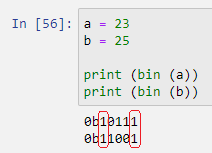by author

The binary value of 23 is 10111, while the binary value of 25 is 11001. So if we position these two binary values one on top of the other, we see that the first and last bit is for both numbers, 1. Meaning, the bitwise and will return 10001, or in decimal, 17.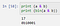by author

It’s important to notice that a bitwise operation returns a decimal value, of the result. For convenience, we use bin() to check the binary value.

Similarly as the logical or, the bitwise or (|) returns a 1 if at least one of the values is 1. Again, if we look at the binary values of 23 and 25, 10111 and 11001, respectively, we see that at least one 1 appears on each position (each bit). And the decimal value of the binary 11111 is 31.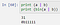by author

The logical XOR operator (^) copies the bit if it’s set in ONLY one operand, but not both. It’s somewhat similar to bitwise or, but in the case of both bits being 1, it will not activate and set the result to 1.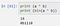by author

The first bit is not printed, since it is zero (0). So the result is basically 01110.

# Membership operators

Membership operators test for membership in a sequence, such as strings, lists, or tuples. I.e., if our goal is to test if an element is contained in a list, tuple, or string. There are two identity operators, in and not in.

IN evaluates if the variable is contained in the desired sequence, string, list, dictionary…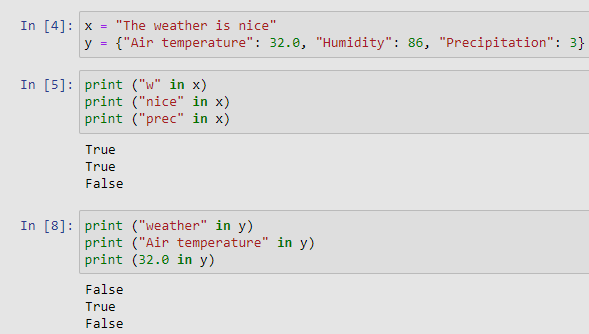by author

Similarly, we use not in, which returns True is the variable IS NOT in the sequence and False if the variable IS IN the sequence.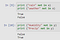by author

# Identity Operators

Identity operators compare the memory locations of certain objects, meaning they do not test if the two objects are equal in value, or size, moreover they test if two objects have the same location in the memory. The identity operators are is and is not.

IS returns True if the objects on the left and right of the operator point to the same object in memory.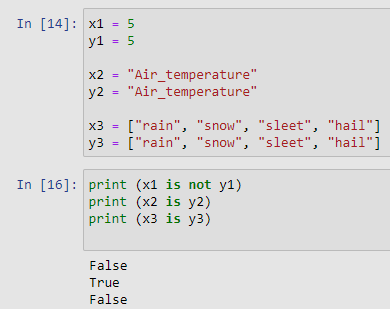by author

Since list are mutable objects, meaning, elements can be added, or elements can be removed from the list using the assignment statement. The examples x1, y1, and x2, y2 contain integers and string, respectively, which are immutable, meaning they once assigned, can not be changed, therefore they also share the same place in the memory.

# Conclusion

With the short article, my goal was to clarify a very important building block of every script or program we write, namely operators. They are certainly not as spectacular as some cool task in deep learning or script to make some colorful plots, but they are a crucial part of every deep learning algorithm or any other task we perform in Python.

I think that we all should invest some time when starting with something new, to understand the basic concepts, since, later, this will make our life much easier.

For any questions or suggestions regarding this article or my other articles on Medium, feel free to contact me via LinkedIn.

Thank you for taking the time, Cheers! 🙂

## Geek Culture

Proud to geek out. Follow to join our +500K monthly readers.

Written by

## Karlo Leskovar

PhD student → Hydrology & Deep Learning. Teaching and Research Assistant at University of Zagreb.

## Geek Culture

A new tech publication by Start it up (https://medium.com/swlh).

Written by

## Karlo Leskovar

PhD student → Hydrology & Deep Learning. Teaching and Research Assistant at University of Zagreb.## Geek Culture

A new tech publication by Start it up (https://medium.com/swlh).

## More From Medium

Medium is an open platform where 170 million readers come to find insightful and dynamic thinking. Here, expert and undiscovered voices alike dive into the heart of any topic and bring new ideas to the surface. Learn more

Follow the writers, publications, and topics that matter to you, and you’ll see them on your homepage and in your inbox. Explore

If you have a story to tell, knowledge to share, or a perspective to offer — welcome home. It’s easy and free to post your thinking on any topic. Write on Medium